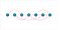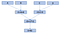Topological sort of a directed acyclic graph [DAG] is partial ordering of its nodes such that U < V implies there must not exist a path from V to U.

Kahn’s algorithm I implemented, instead produces a linear ordering such that […, U, …, V, …] means there may be a path from U to V, but not vice versa.topological sort: all edges are directed from left to right

Partial ordering is very useful in many situations. One of them arises in parallel computing where a program can be represented as DAG.

`E = (A + B) * (C - D)`

Each node represents an operation and directed links in between are their dependencies.topological sort of DAG representing the expression

There are 8 operations [including fetch and store] in this expression, but modern super-scalar CPUs are able to execute some operations in parallel to reduce the execution time up to 4 steps.

https://github.com/coells/100days

https://notebooks.azure.com/coells/libraries/100days

## algorithm

`def topological_sort(graph):    topology = []    degree = {n: graph.in_degree(n) for n in graph.nodes()}    # nodes without incoming edges    queue = deque(n for n, d in degree.items() if not d)        while queue:        n = queue.popleft()        topology.append(n)        # remove node's edges        for m in list(graph[n]):            degree[m] -= 1            # enqueue nodes with no incoming edges            if not degree[m]:                queue.append(m)    if len(topology) < len(graph):        raise ValueError('graph contains cycle')    return topology`

## run

`graph = nx.DiGraph([    ('A', 'B'),    ('A', 'D'),    ('B', 'C'),    ('B', 'E'),    ('C', 'D'),    ('C', 'E'),    ('D', 'E'),    ('F', 'G'),])topological_sort(graph)>>>['A', 'F', 'B', 'G', 'C', 'D', 'E']`

Written by

## 100 days of algorithms

#### 100 days, 100 algorithms - a challenge consisting of many small pieces

Welcome to a place where words matter. On Medium, smart voices and original ideas take center stage - with no ads in sight. Watch
Follow all the topics you care about, and we’ll deliver the best stories for you to your homepage and inbox. Explore
Get unlimited access to the best stories on Medium — and support writers while you’re at it. Just \$5/month. Upgrade